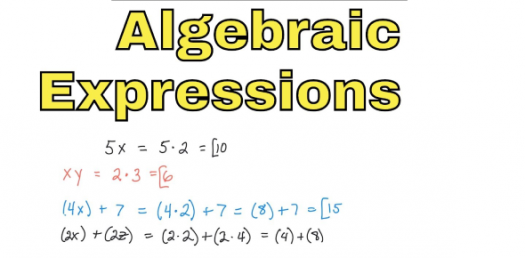# Algebraic Expressions Trivia Questions Test! Quiz

15 Questions | Total Attempts: 105SettingsThe quiz below is made up of a set of algebraic expressions in which a student is expected to either simply the equation or solve it. The quiz is for many of us who have some challenges in this topic. Take it up and begin slow first before you tackle the other algebra quizzes on this site. All the best!

• 1.
Give one way to write each algebraic expression in words.  Use numbers to represent words and use words to represent symbols.
• 2.
Give one way to write each algebraic expression in words.  Use numbers to represent words and use words to represent symbols.
• 3.
Give one way to write each algebraic expression in words.  Use numbers to represent words and use words to represent symbols.
• 4.
Give one way to write each algebraic expression in words.  Use numbers to represent words and use words to represent symbols.
• 5.
Give one way to write each algebraic expression in words.  Use numbers to represent words and use words to represent symbols.
• 6.
Give one way to write each algebraic expression in words.  Use numbers to represent words and use words to represent symbols.
• 7.
Use letters, numbers and symbols to answer the following question:
• 8.
Use letters, numbers and symbols to answer the following question:
• 9.
• 10.
• 11.
• 12.
• 13.
• 14.
• 15.
Submit your answer in the following form:Answer for part A; Answer for part B.  Note that a semicolon (;) and space separate the two answers.  Leave no spaces between the variable (letter) the symbol and the number you use.  For part B put your answers in as A,B,C with no spaces and replacing the letters with the appropriate numbers.
Related TopicsBack to top